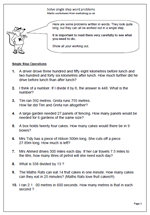# Year 5 maths worksheet: solve single step word problemsThis page contains a set of ten problems written in words. They look quite long, but they can all be worked out using a single step, using one of the operations: addition, subtraction, multiplication and division. Because they only require one step they are easier than questions which involve two or steps, but each calculation is quite tricky, with several needing a good knowledge of written methods to be able to do them.

It is important that all working out is shown for several reasons, including to show that a calculator was not used and to be able to spot where errors occur. Wrong answers are an excellent step towards new learning!

It is also interesting to see how children approach the questions. One or two questions in particular may cause problems. Question 6, involves subtracting of decimals and can be laid out in the standard format but could also be answered by adding on: e.g.

27.89 + 0.11 = 28

28 + 72 = 100

100 + 200 = 300

answer = 0.11 + 72 + 200 = 272.11

Solve single step word problems